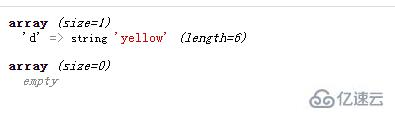# php怎么比较两个数组是否相等• 如果两个数组的差集为空，则这两个数组中没有不同的元素，即两个数组是相等的

• 如果两个数组的差集不为空，则这两个数组中有不同的元素，即两个数组是不相等的

• array_diff_assoc()用于比较两个（或更多个）数组的键名和键值 ，并返回差集数组。

1、使用array_diff_assoc()获取数组差集

```<?php
\$arr1=array("a"=>"red","b"=>"green","c"=>"blue","d"=>"yellow");
\$arr2=array("a"=>"red","b"=>"green","c"=>"blue");
\$arr3=array("a"=>"red","b"=>"green","c"=>"blue");
\$diff1=array_diff_assoc(\$arr1,\$arr2);
var_dump(\$diff1);
\$diff2=array_diff_assoc(\$arr2,\$arr3);
var_dump(\$diff2);
?>```2、判断差集数组是否为空数组

```if(\$diff1==[]){
echo "两数组相等<br>";
}
else{
echo "两数组不相等<br>";
}``````if(\$diff2==[]){
echo "两数组相等<br>";
}
else{
echo "两数组不相等<br>";
}``````<?php
function f(\$arr1,\$arr2){
\$diff1=array_diff_assoc(\$arr1,\$arr2);
if(\$diff1==[]){
echo "两数组相等<br>";
}
else{
echo "两数组不相等<br>";
}
}

\$arr1=array("a"=>"red","b"=>"green","c"=>"blue","d"=>"yellow");
\$arr2=array("a"=>"red","b"=>"green","c"=>"blue");
\$arr3=array("a"=>"red","b"=>"green","c"=>"blue");

f(\$arr1,\$arr2);

f(\$arr2,\$arr3);
?>```